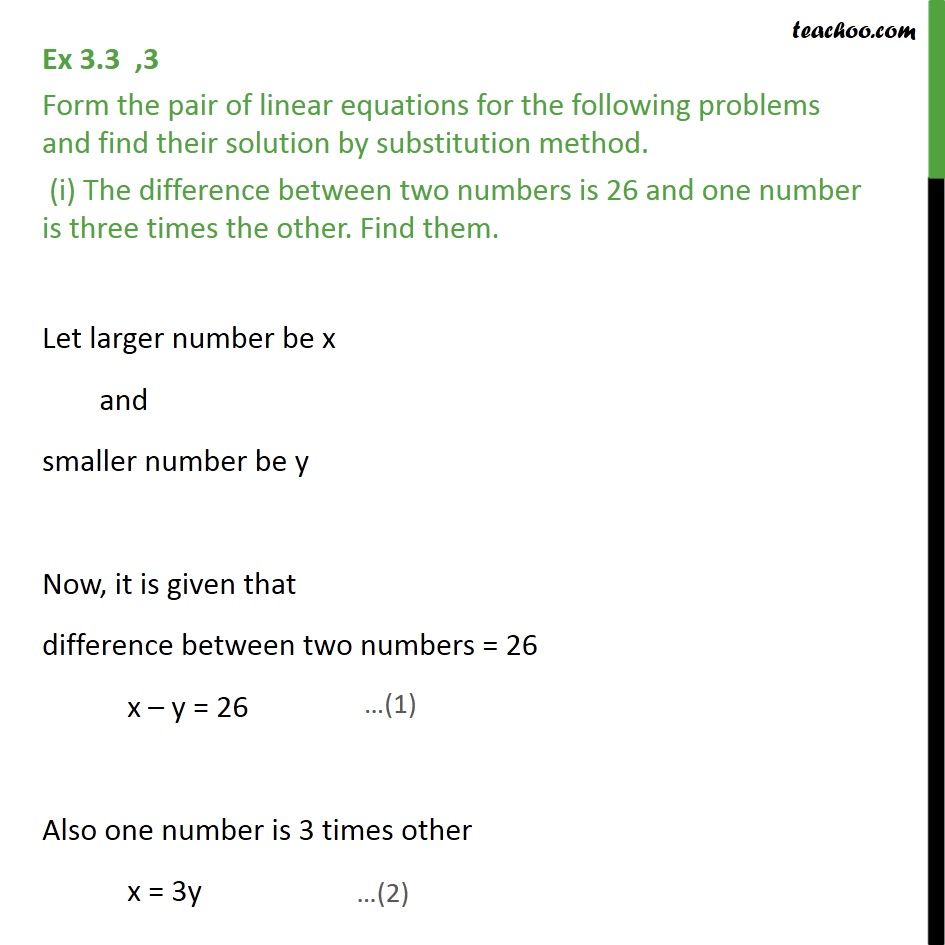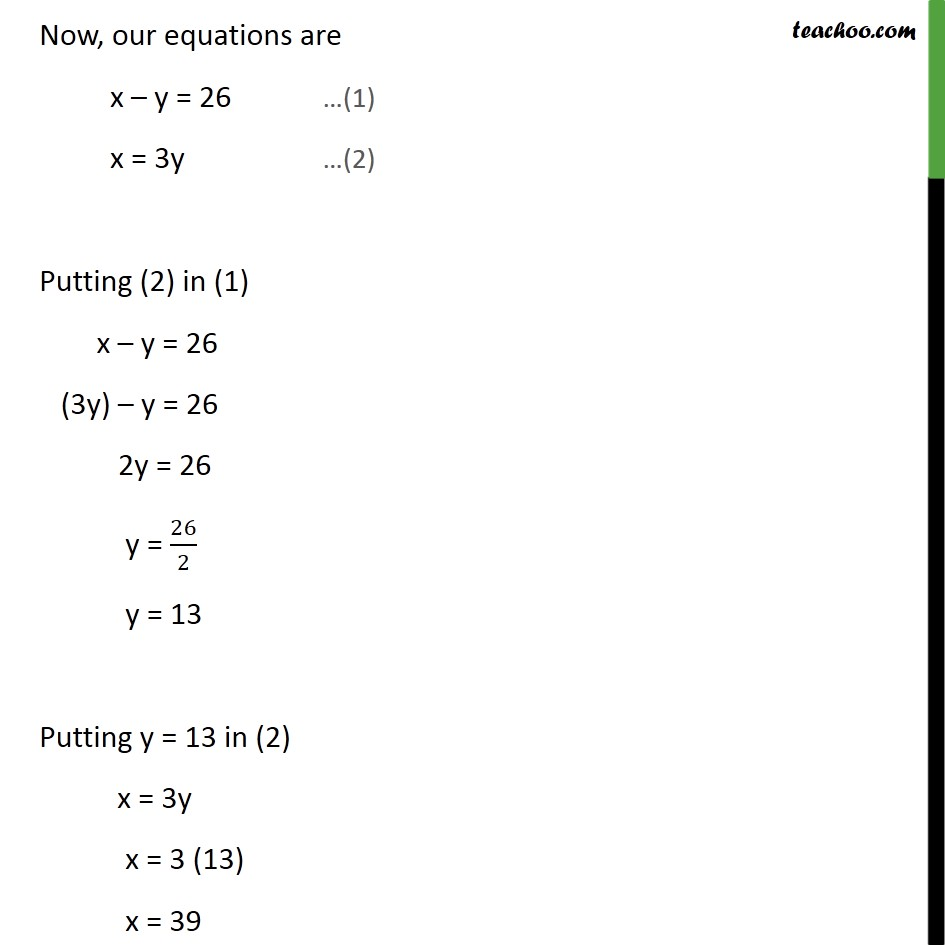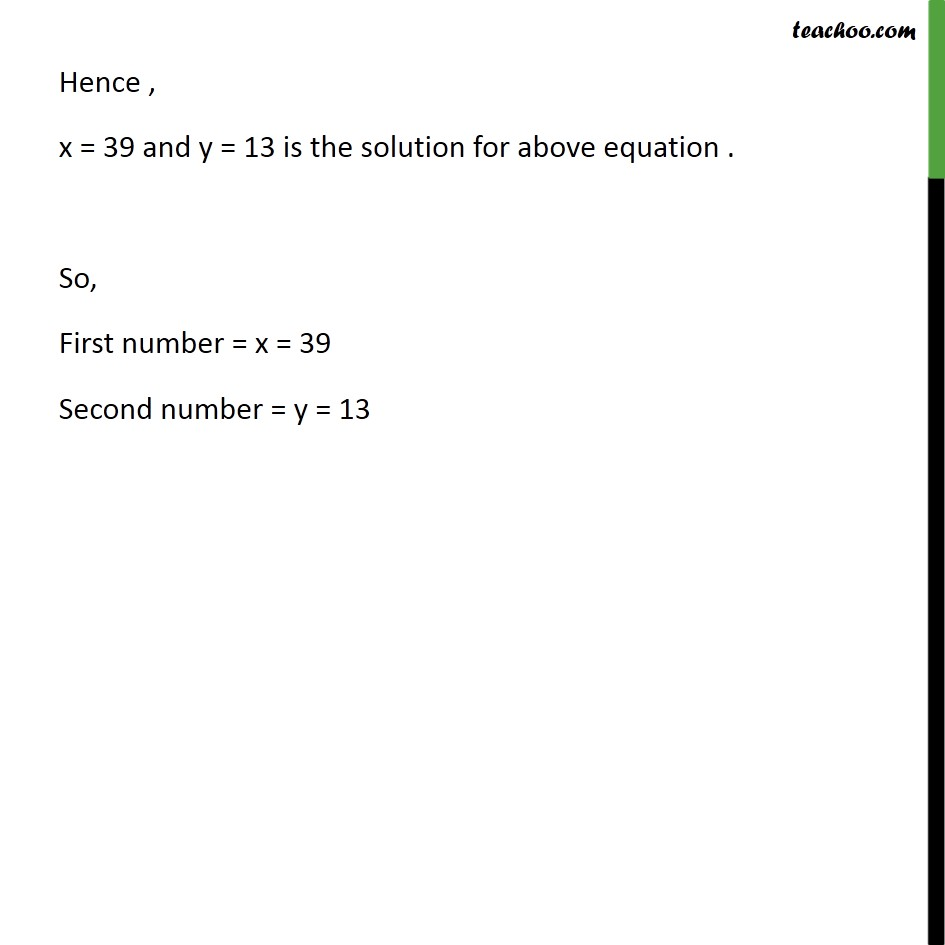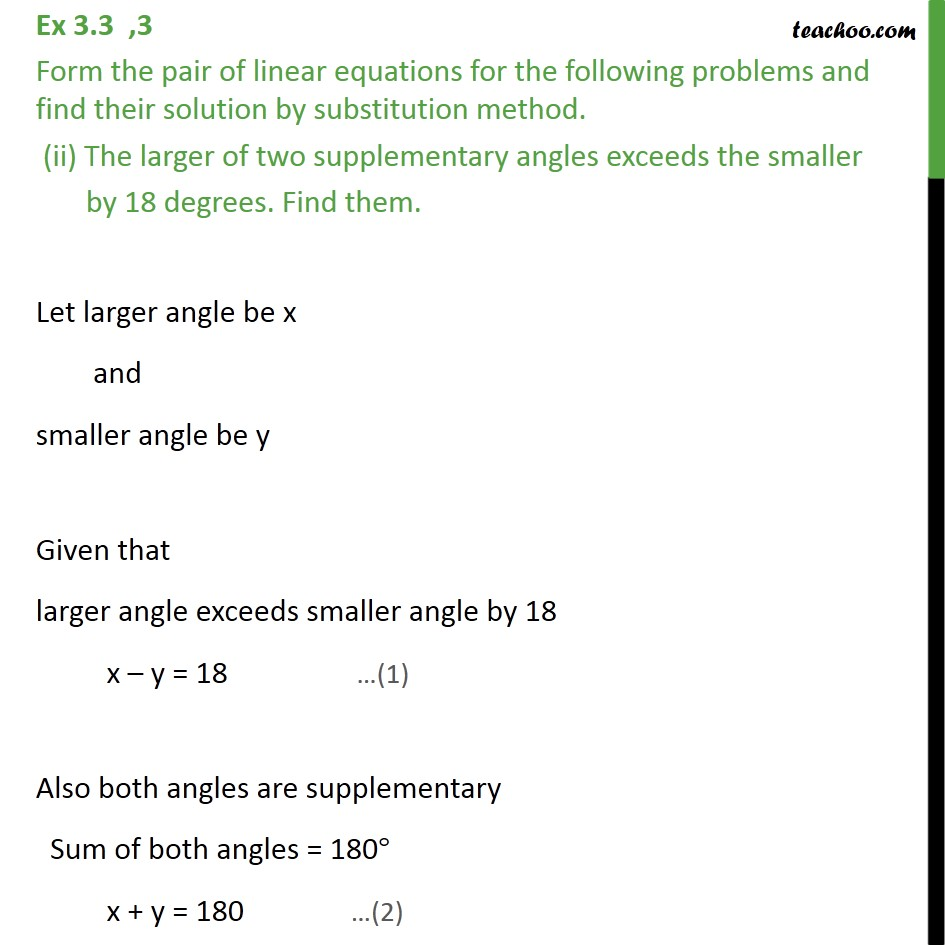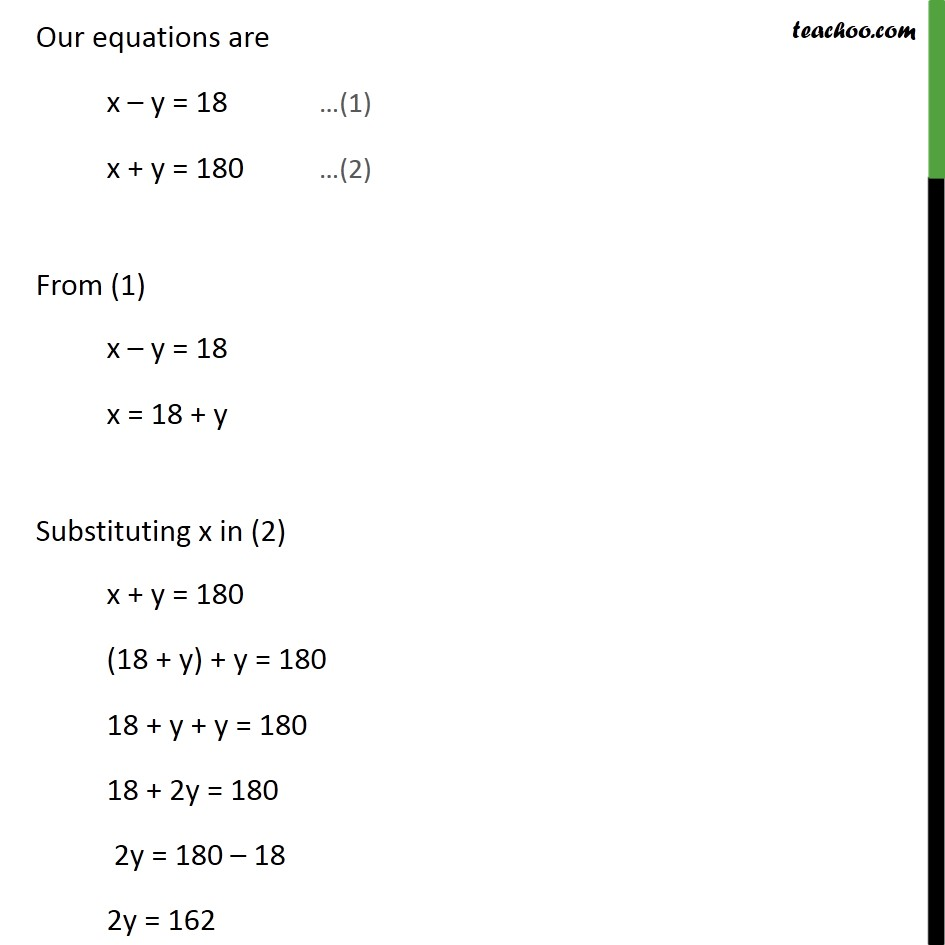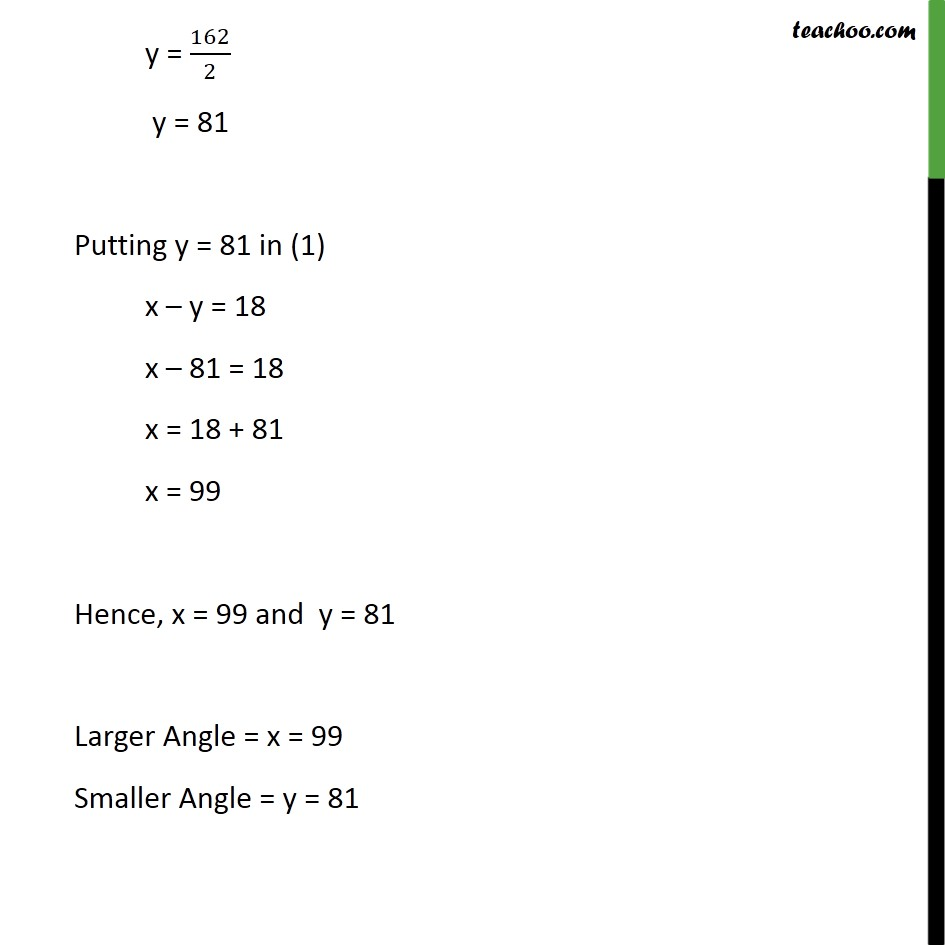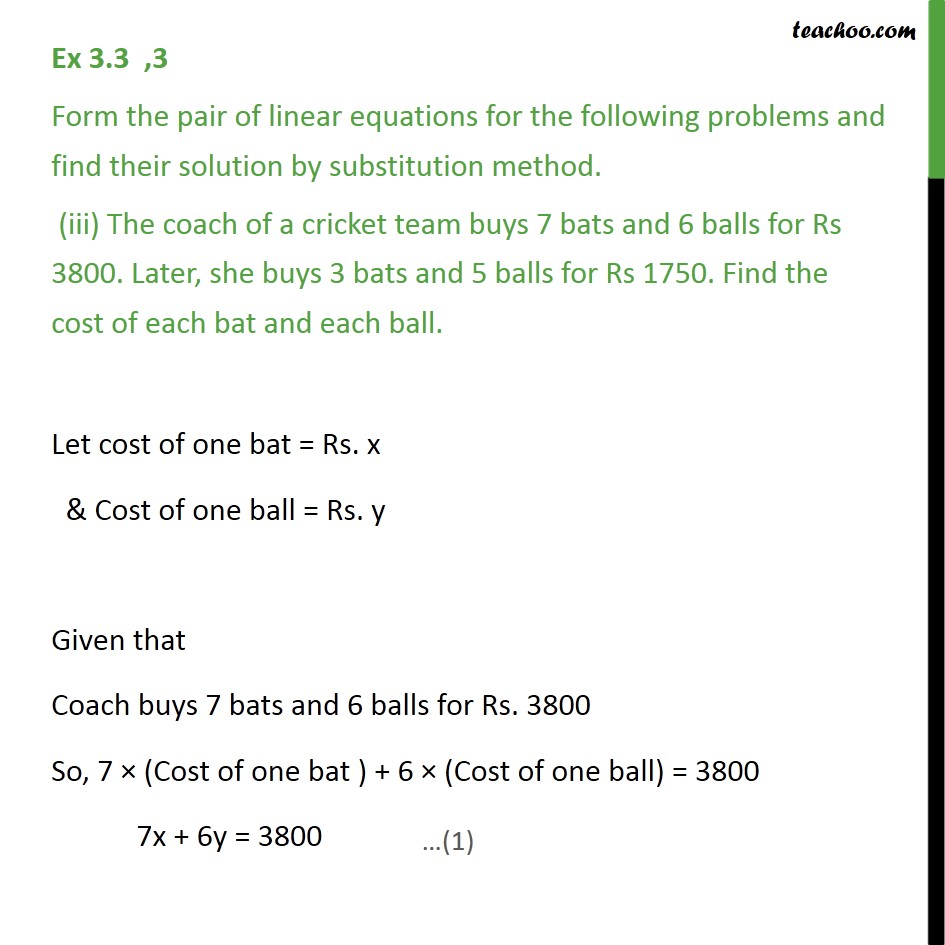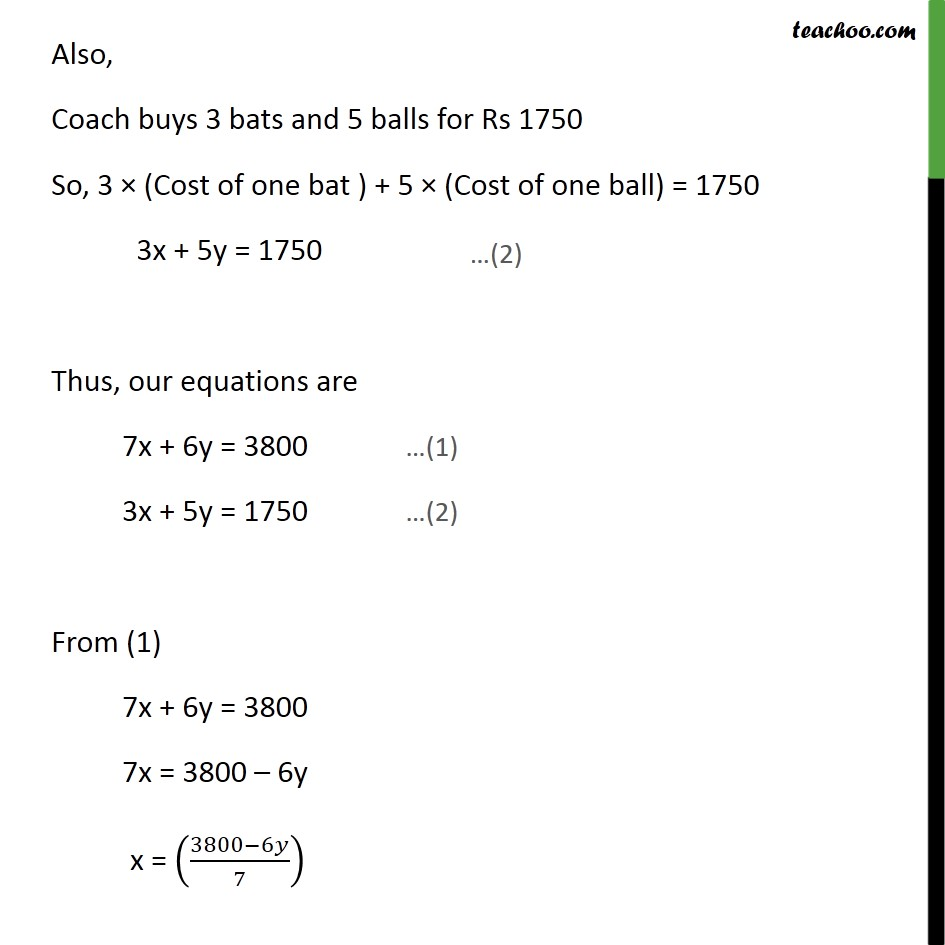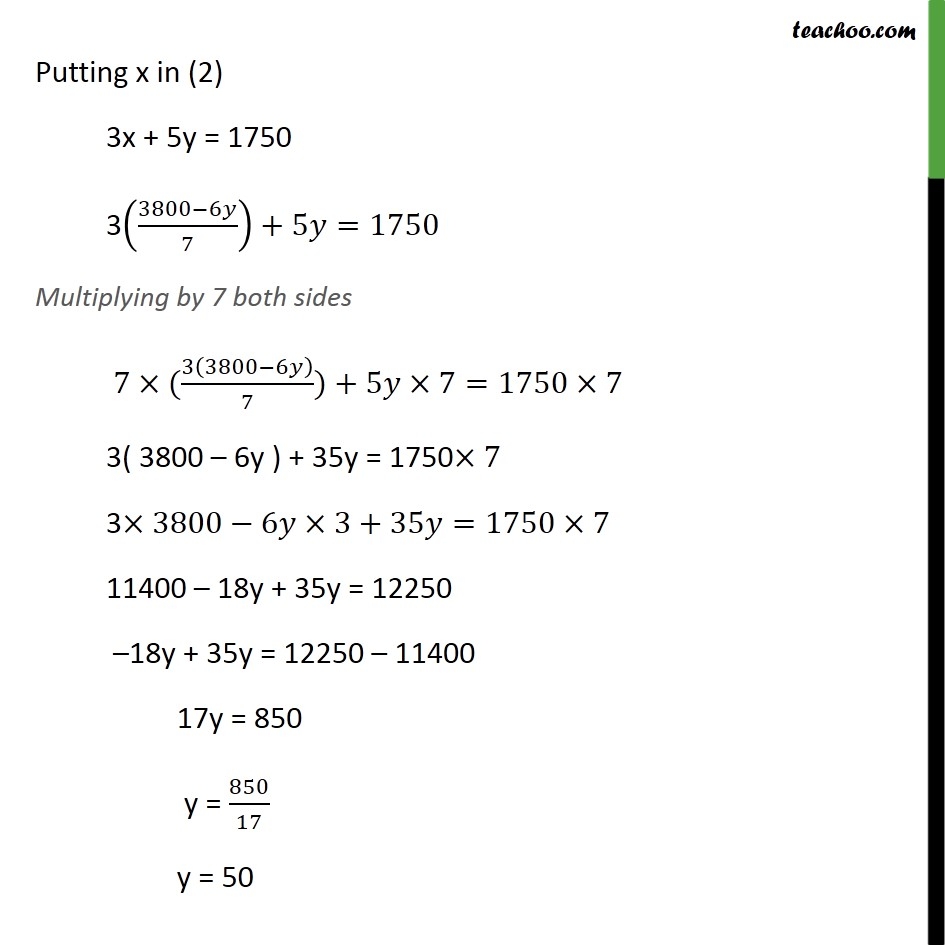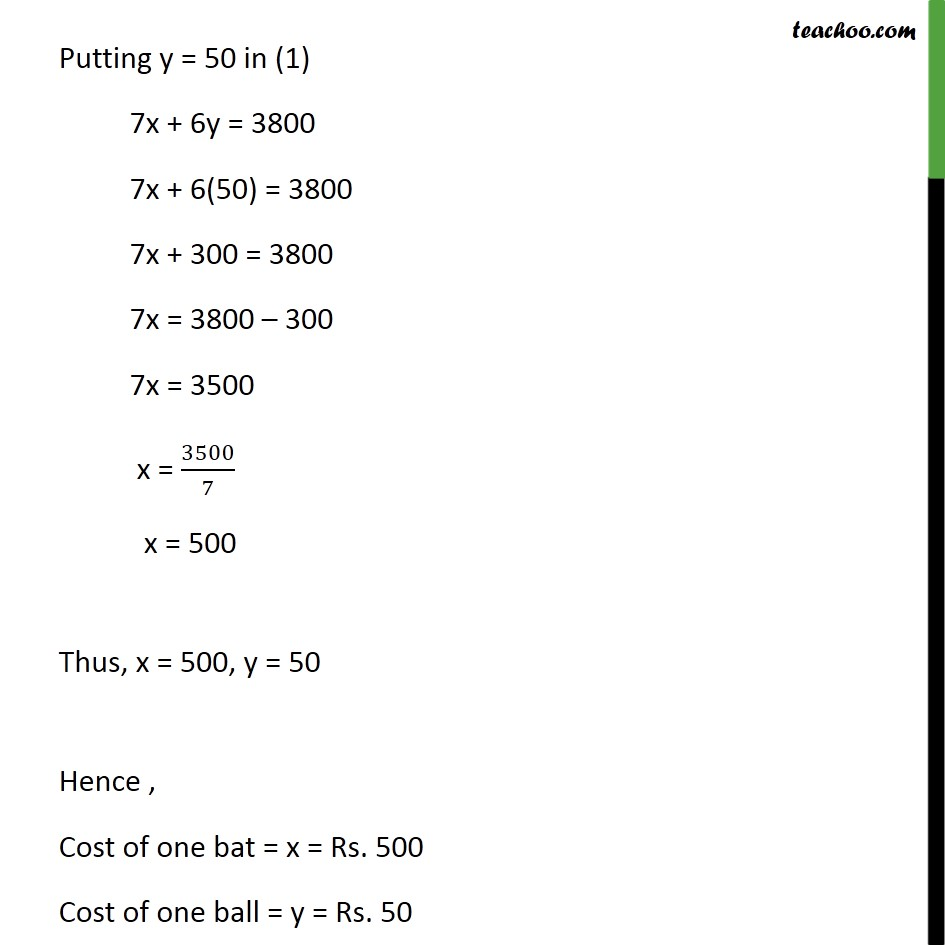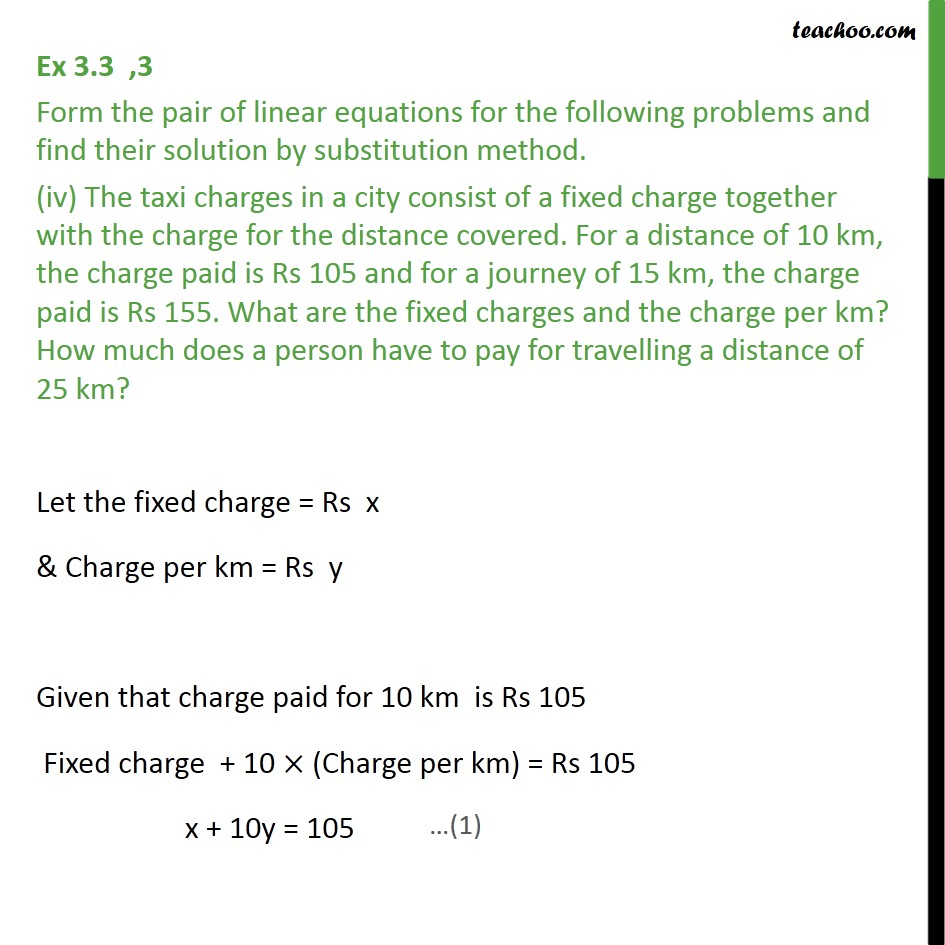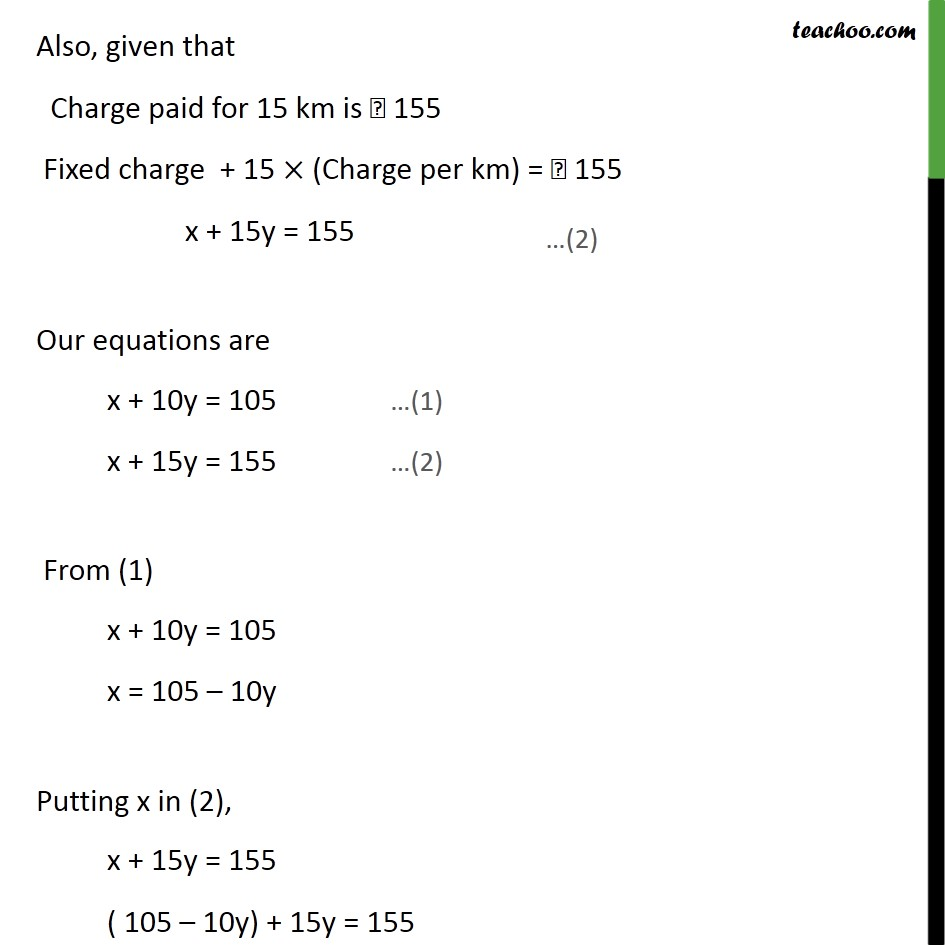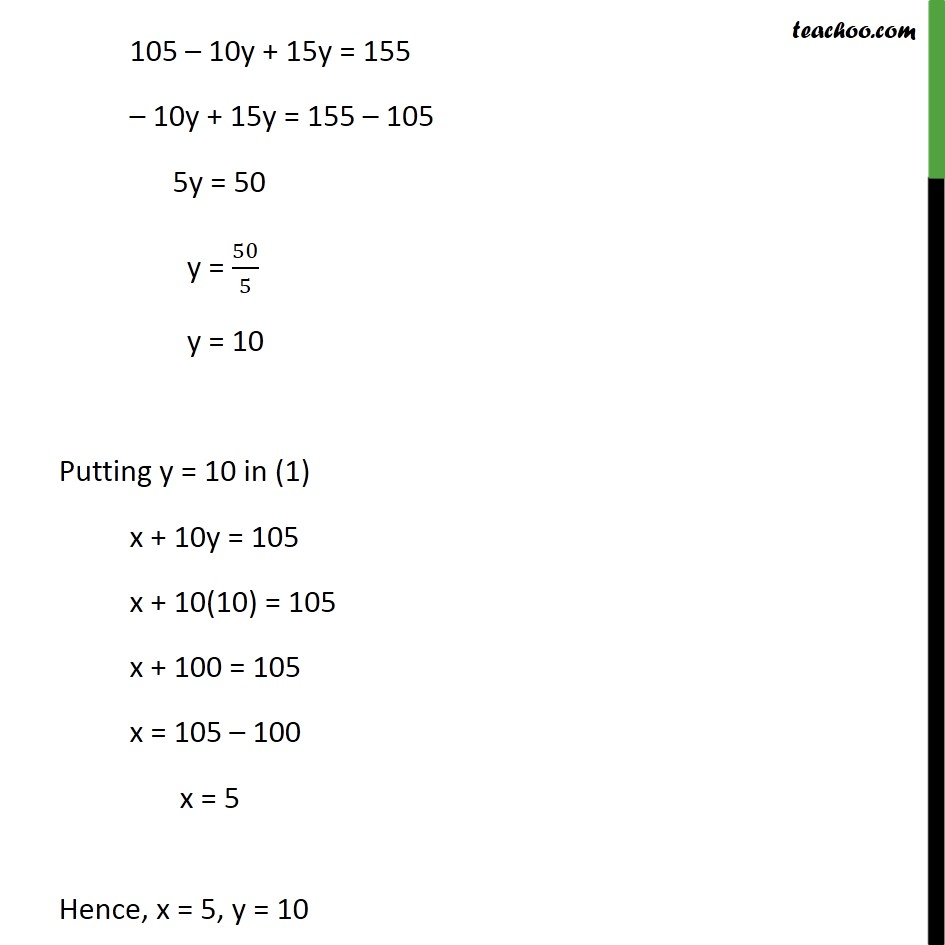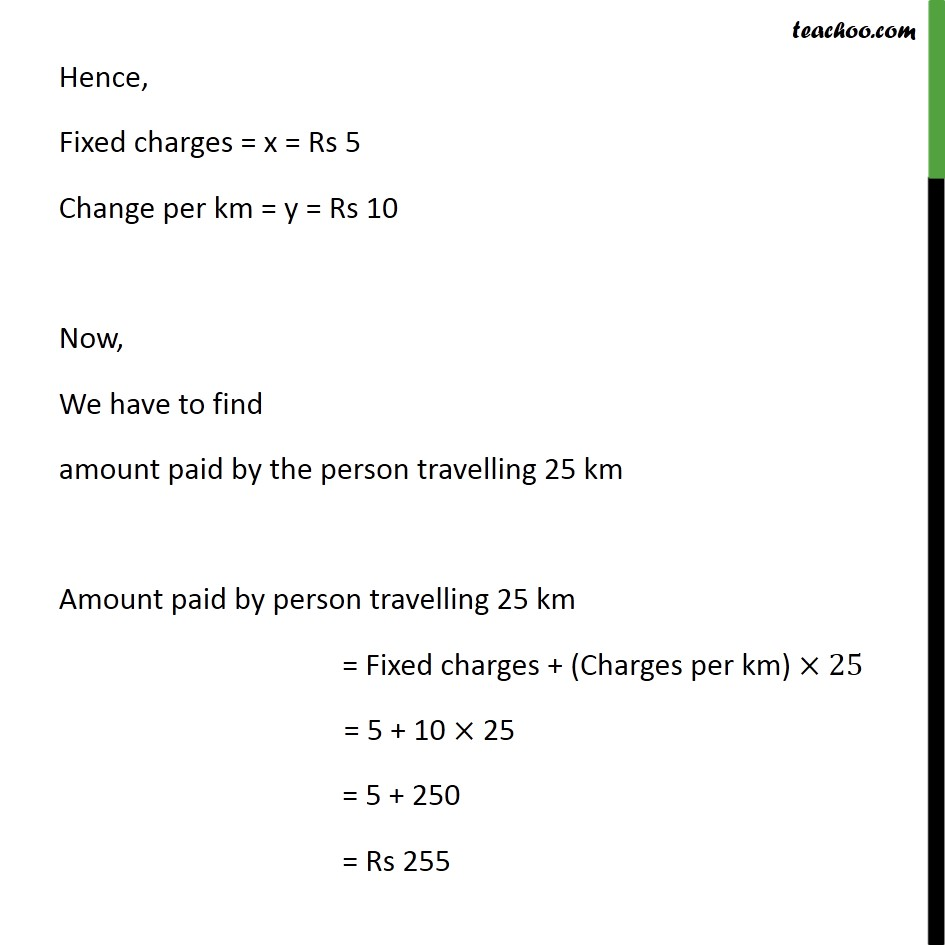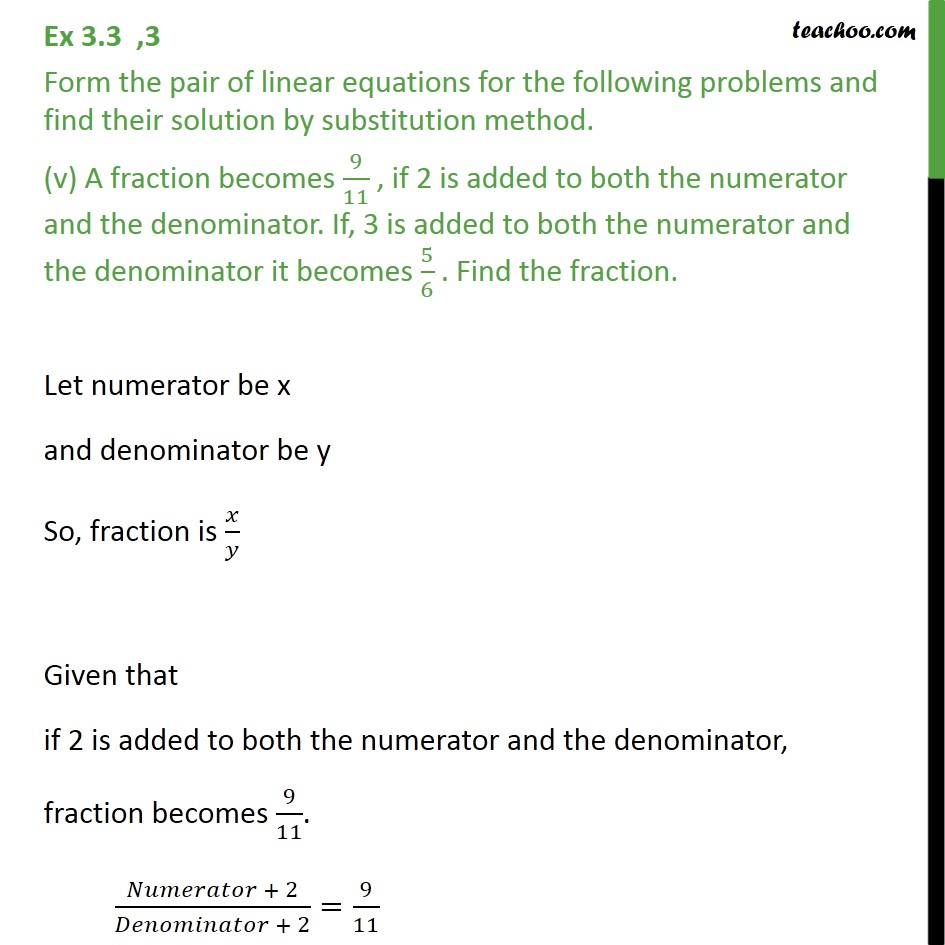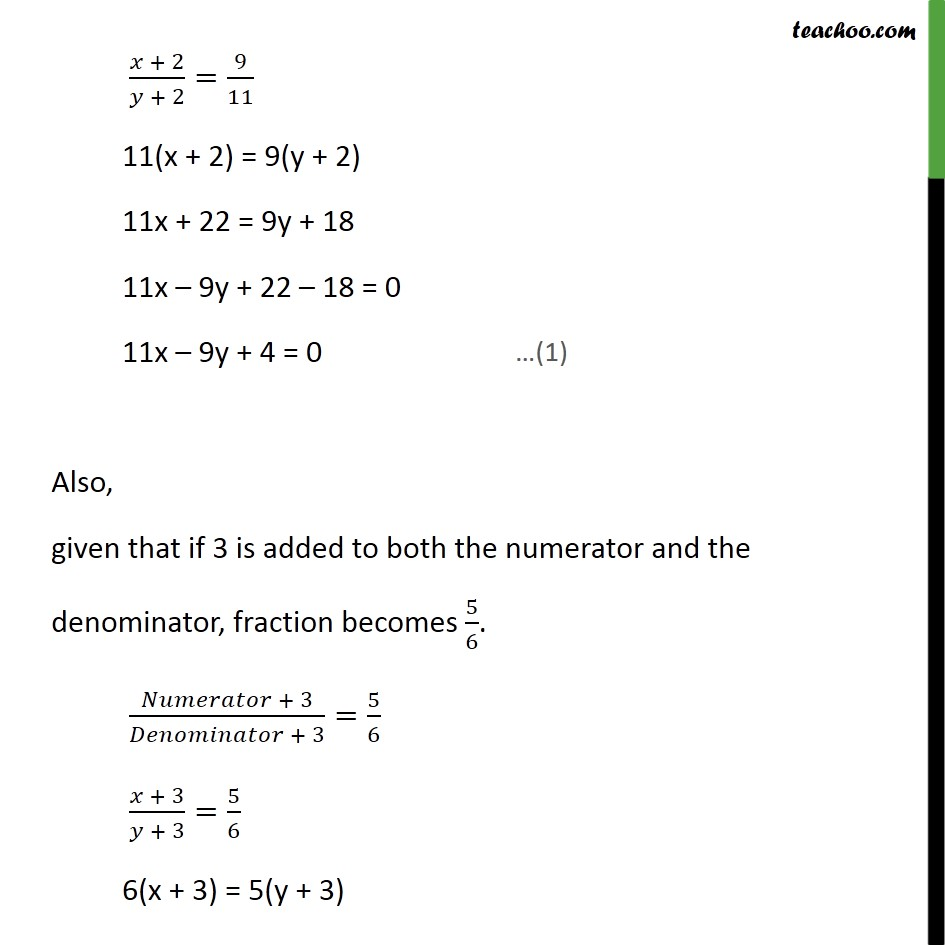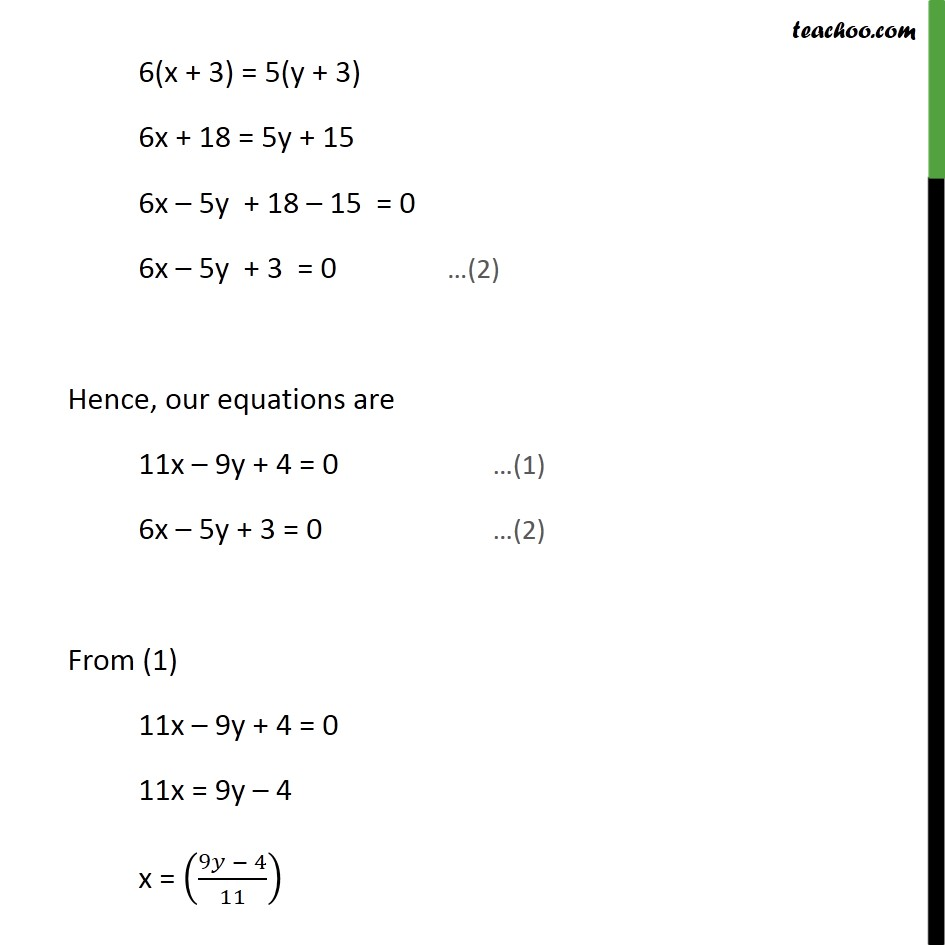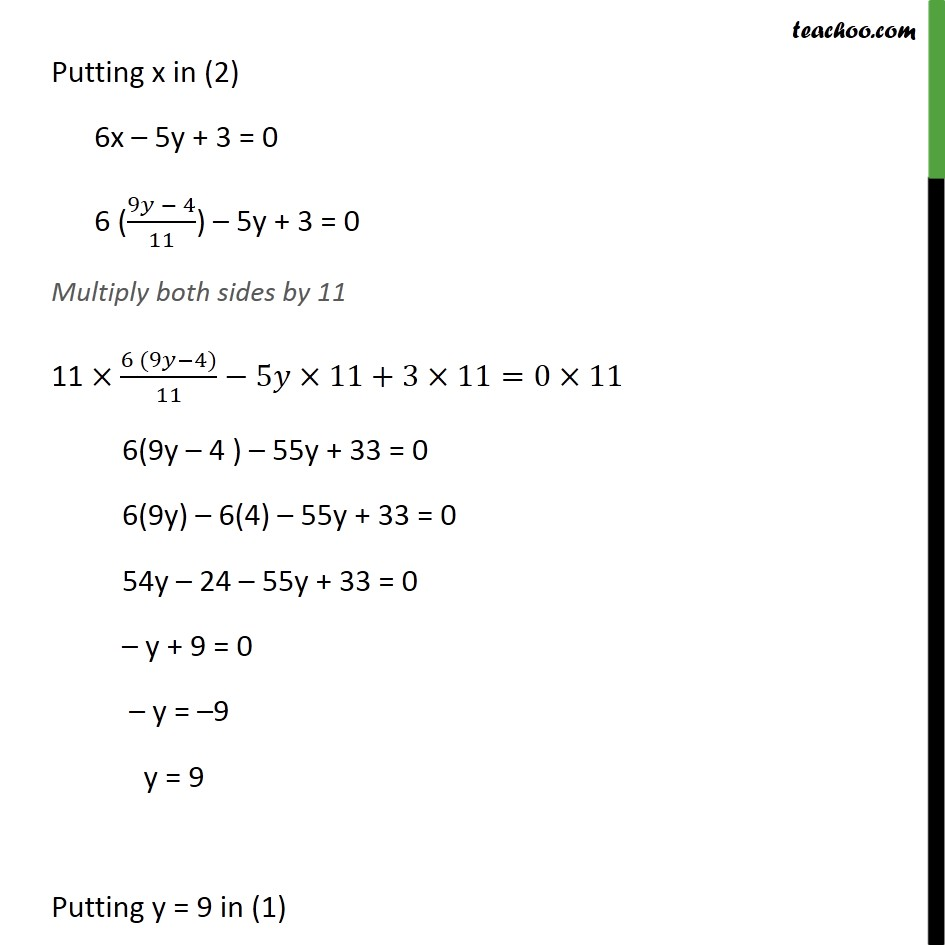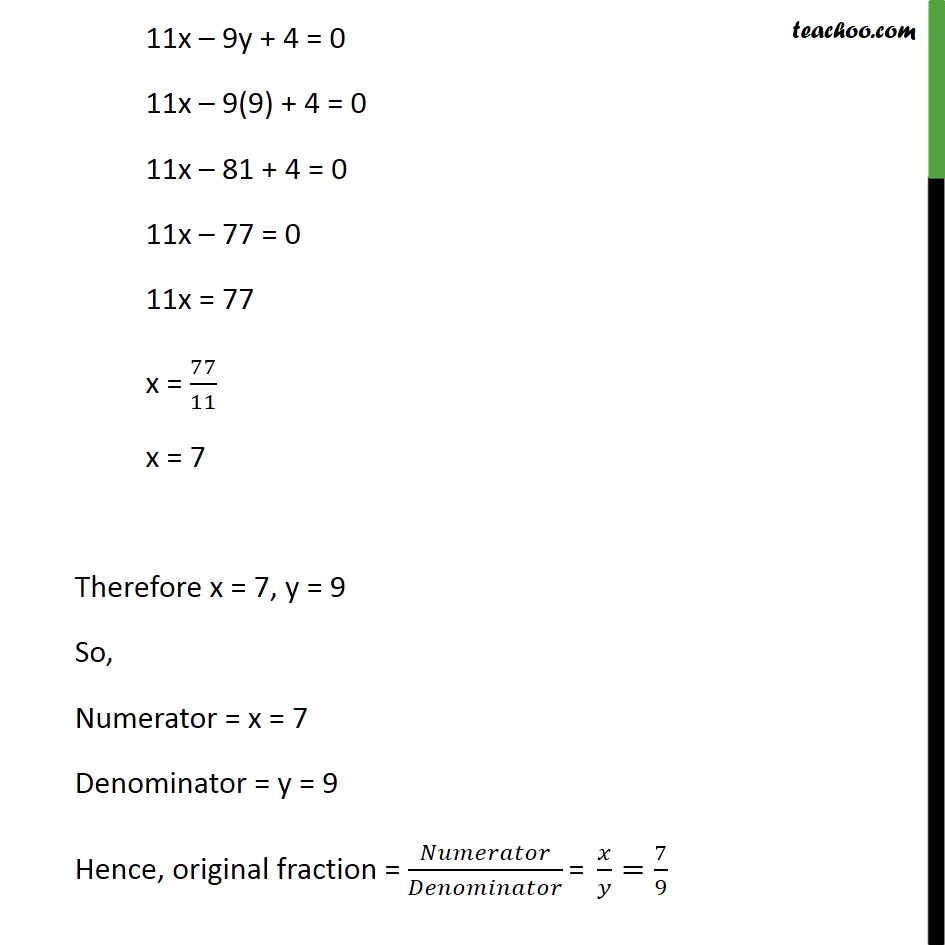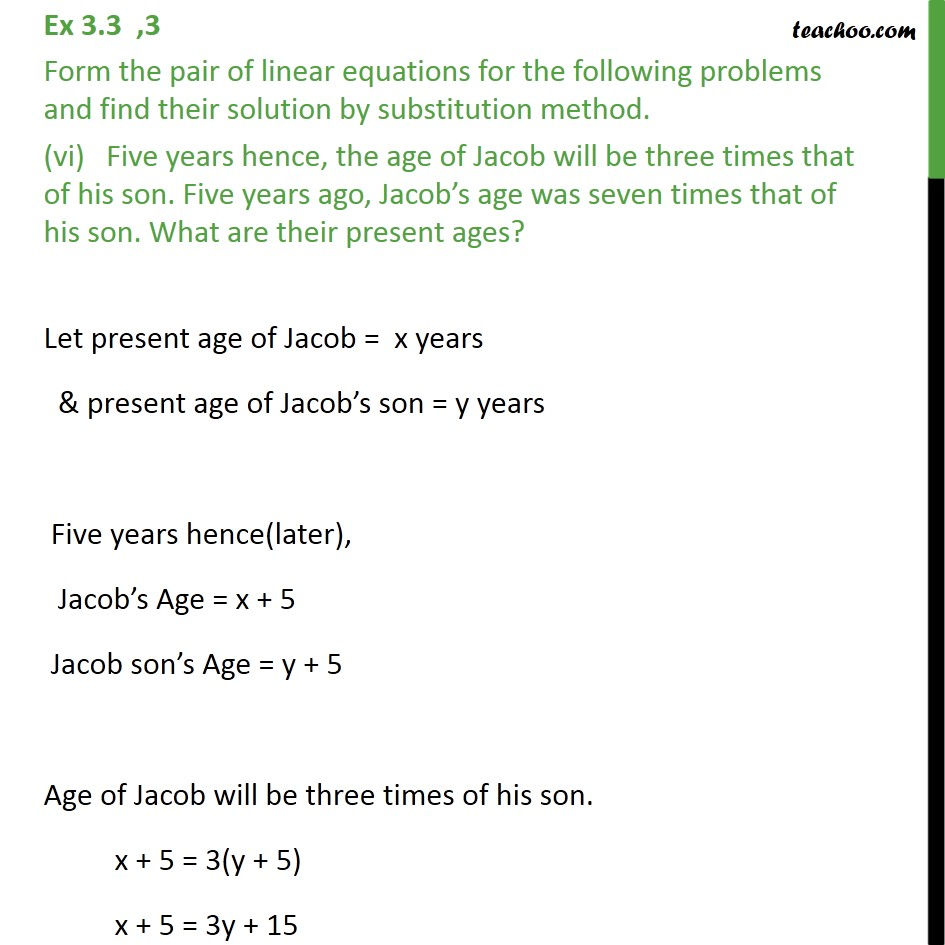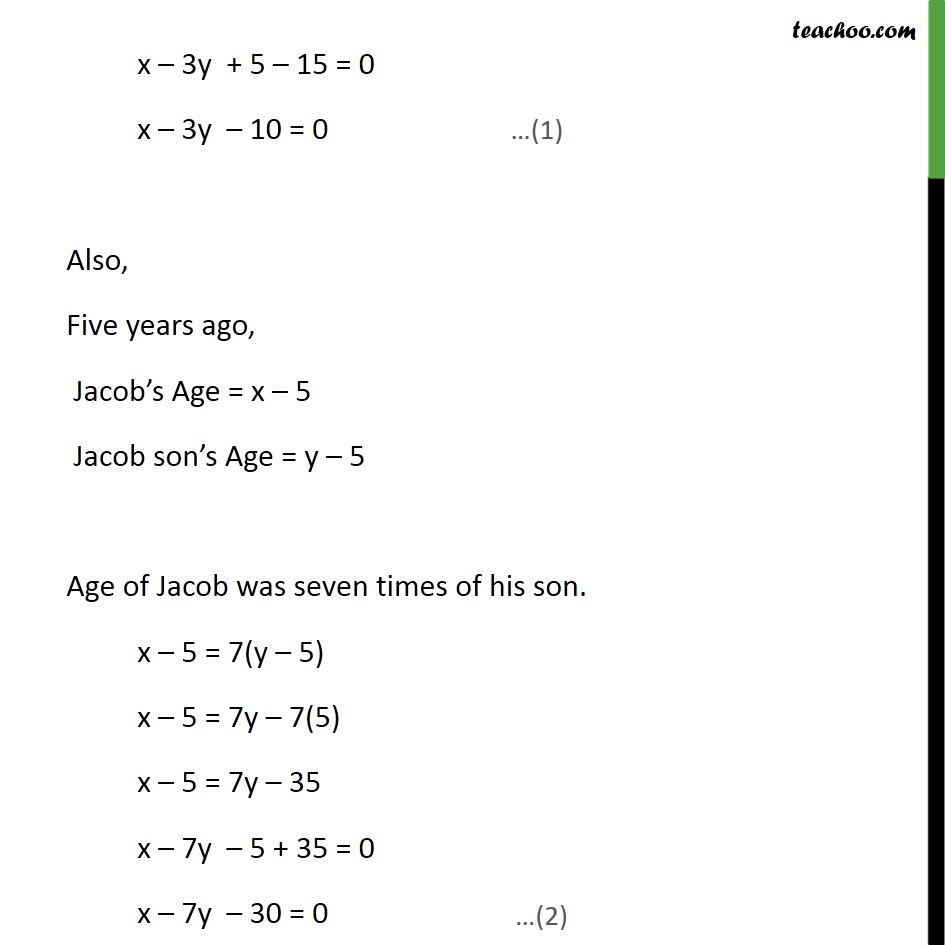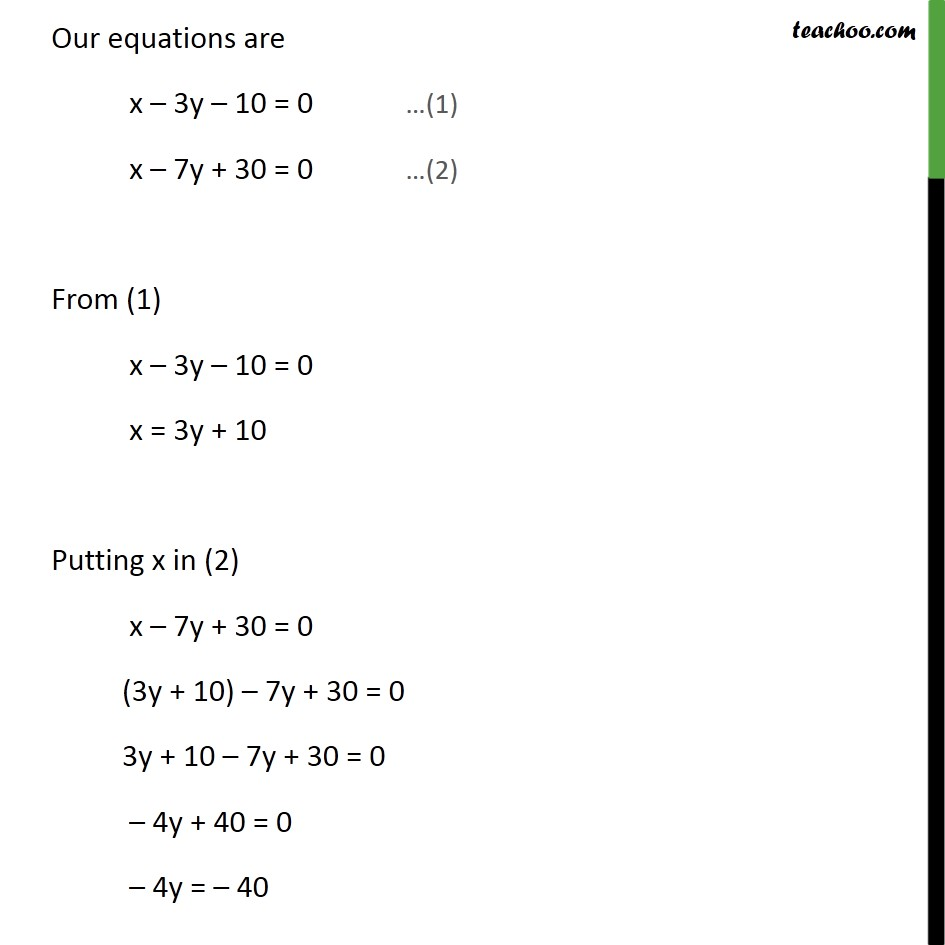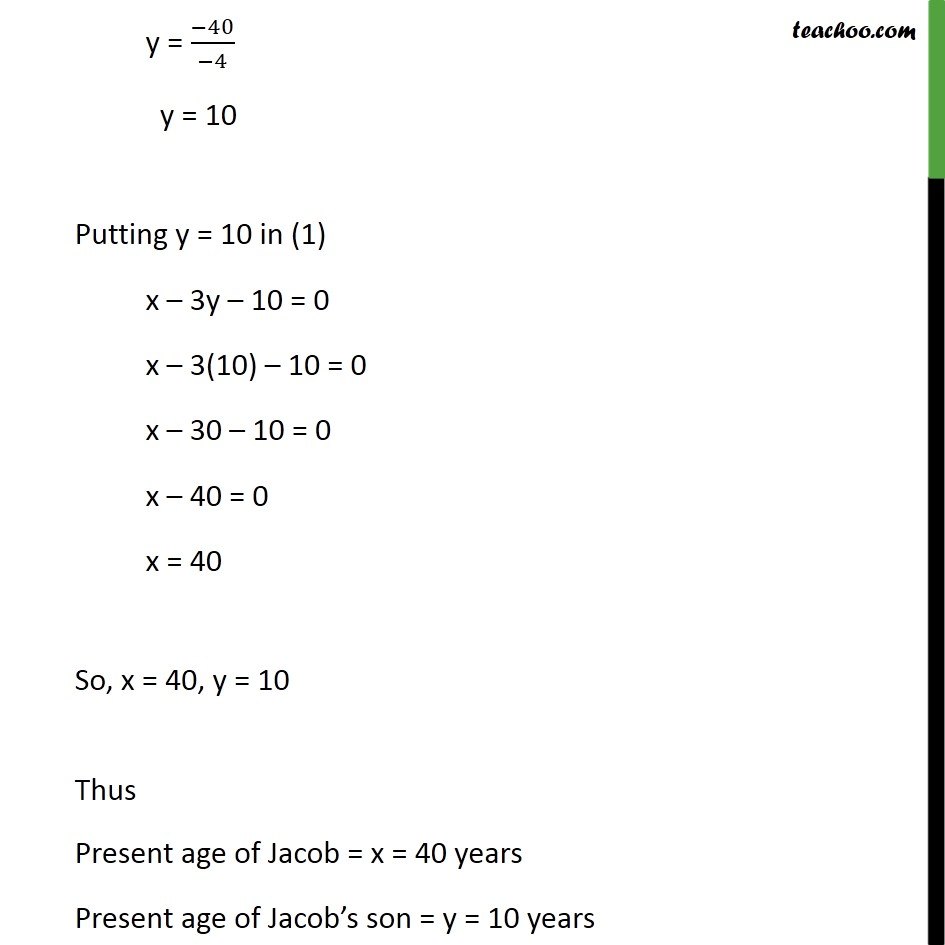1. Chapter 3 Class 10 Pair of Linear Equations in Two Variables
2. Serial order wise
3. Ex 3.3

Transcript

Ex 3.3 ,3 Form the pair of linear equations for the following problems and find their solution by substitution method. (i) The difference between two numbers is 26 and one number is three times the other. Find them. Let larger number be x and smaller number be y Now, it is given that difference between two numbers = 26 x – y = 26 Also one number is 3 times other x = 3y Now, our equations are x – y = 26 …(1) x = 3y …(2) Putting (2) in (1) x – y = 26 (3y) – y = 26 2y = 26 y = 26/2 y = 13 Putting y = 13 in (2) x = 3y x = 3 (13) x = 39 Hence , x = 39 and y = 13 is the solution for above equation . So, First number = x = 39 Second number = y = 13 Ex 3.3 ,3 Form the pair of linear equations for the following problems and find their solution by substitution method. (ii) The larger of two supplementary angles exceeds the smaller by 18 degrees. Find them. Let larger angle be x and smaller angle be y Given that larger angle exceeds smaller angle by 18 x – y = 18 Also both angles are supplementary Sum of both angles = 180° x + y = 180 Our equations are x – y = 18 …(1) x + y = 180 …(2) From (1) x – y = 18 x = 18 + y Substituting x in (2) x + y = 180 (18 + y) + y = 180 18 + y + y = 180 18 + 2y = 180 2y = 180 – 18 2y = 162 y = 162/2 y = 81 Putting y = 81 in (1) x – y = 18 x – 81 = 18 x = 18 + 81 x = 99 Hence, x = 99 and y = 81 Larger Angle = x = 99 Smaller Angle = y = 81 Ex 3.3 ,3 Form the pair of linear equations for the following problems and find their solution by substitution method. (iii) The coach of a cricket team buys 7 bats and 6 balls for Rs 3800. Later, she buys 3 bats and 5 balls for Rs 1750. Find the cost of each bat and each ball. Let cost of one bat = Rs. x & Cost of one ball = Rs. y Given that Coach buys 7 bats and 6 balls for Rs. 3800 So, 7 × (Cost of one bat ) + 6 × (Cost of one ball) = 3800 7x + 6y = 3800 Also, Coach buys 3 bats and 5 balls for Rs 1750 So, 3 × (Cost of one bat ) + 5 × (Cost of one ball) = 1750 3x + 5y = 1750 Thus, our equations are 7x + 6y = 3800 …(1) 3x + 5y = 1750 …(2) From (1) 7x + 6y = 3800 7x = 3800 – 6y x = ((3800−6𝑦)/7) Putting x in (2) 3x + 5y = 1750 3((3800−6𝑦)/7)+5𝑦=1750 Multiplying by 7 both sides 7×(3(3800−6𝑦)/7)+5𝑦×7=1750×7 3( 3800 – 6y ) + 35y = 1750×7 3×3800−6𝑦×3+35𝑦=1750×7 11400 – 18y + 35y = 12250 –18y + 35y = 12250 – 11400 17y = 850 y = 850/17 y = 50 Putting y = 50 in (1) 7x + 6y = 3800 7x + 6(50) = 3800 7x + 300 = 3800 7x = 3800 – 300 7x = 3500 x = 3500/7 x = 500 Thus, x = 500, y = 50 Hence , Cost of one bat = x = Rs. 500 Cost of one ball = y = Rs. 50 Ex 3.3 ,3 Form the pair of linear equations for the following problems and find their solution by substitution method. (iv) The taxi charges in a city consist of a fixed charge together with the charge for the distance covered. For a distance of 10 km, the charge paid is Rs 105 and for a journey of 15 km, the charge paid is Rs 155. What are the fixed charges and the charge per km? How much does a person have to pay for travelling a distance of 25 km? Let the fixed charge = Rs x & Charge per km = Rs y Given that charge paid for 10 km is Rs 105 Fixed charge + 10 × (Charge per km) = Rs 105 x + 10y = 105 Also, given that Charge paid for 15 km is ₹ 155 Fixed charge + 15 × (Charge per km) = ₹ 155 x + 15y = 155 Our equations are x + 10y = 105 …(1) x + 15y = 155 …(2) From (1) x + 10y = 105 x = 105 – 10y Putting x in (2), x + 15y = 155 ( 105 – 10y) + 15y = 155 105 – 10y + 15y = 155 – 10y + 15y = 155 – 105 5y = 50 y = 50/5 y = 10 Putting y = 10 in (1) x + 10y = 105 x + 10(10) = 105 x + 100 = 105 x = 105 – 100 x = 5 Hence, x = 5, y = 10 Hence, Fixed charges = x = Rs 5 Change per km = y = Rs 10 Now, We have to find amount paid by the person travelling 25 km Amount paid by person travelling 25 km = Fixed charges + (Charges per km) ×25 = 5 + 10 × 25 = 5 + 250 = Rs 255 Ex 3.3 ,3 Form the pair of linear equations for the following problems and find their solution by substitution method. (v) A fraction becomes 9/11 , if 2 is added to both the numerator and the denominator. If, 3 is added to both the numerator and the denominator it becomes 5/6 . Find the fraction. Let numerator be x and denominator be y So, fraction is 𝑥/𝑦 Given that if 2 is added to both the numerator and the denominator, fraction becomes 9/11. (𝑁𝑢𝑚𝑒𝑟𝑎𝑡𝑜𝑟 + 2)/(𝐷𝑒𝑛𝑜𝑚𝑖𝑛𝑎𝑡𝑜𝑟 + 2)=9/11 (𝑥 + 2)/(𝑦 + 2)=9/11 11(x + 2) = 9(y + 2) 11x + 22 = 9y + 18 11x – 9y + 22 – 18 = 0 11x – 9y + 4 = 0 Also, given that if 3 is added to both the numerator and the denominator, fraction becomes 5/6. (𝑁𝑢𝑚𝑒𝑟𝑎𝑡𝑜𝑟 + 3)/(𝐷𝑒𝑛𝑜𝑚𝑖𝑛𝑎𝑡𝑜𝑟 + 3)=5/6 (𝑥 + 3)/(𝑦 + 3)=5/6 6(x + 3) = 5(y + 3) 6(x + 3) = 5(y + 3) 6x + 18 = 5y + 15 6x – 5y + 18 – 15 = 0 6x – 5y + 3 = 0 Hence, our equations are 11x – 9y + 4 = 0 …(1) 6x – 5y + 3 = 0 …(2) From (1) 11x – 9y + 4 = 0 11x = 9y – 4 x = ((9𝑦 − 4)/11) Putting x in (2) 6x – 5y + 3 = 0 6 ((9𝑦 − 4)/11) – 5y + 3 = 0 Multiply both sides by 11 11 ×(6 (9𝑦−4))/11−5𝑦×11+3×11=0×11 6(9y – 4 ) – 55y + 33 = 0 6(9y) – 6(4) – 55y + 33 = 0 54y – 24 – 55y + 33 = 0 – y + 9 = 0 – y = –9 y = 9 Putting y = 9 in (1) 11x – 9y + 4 = 0 11x – 9(9) + 4 = 0 11x – 81 + 4 = 0 11x – 77 = 0 11x = 77 x = 77/11 x = 7 Therefore x = 7, y = 9 So, Numerator = x = 7 Denominator = y = 9 Hence, original fraction = 𝑁𝑢𝑚𝑒𝑟𝑎𝑡𝑜𝑟/𝐷𝑒𝑛𝑜𝑚𝑖𝑛𝑎𝑡𝑜𝑟 "= " 𝑥/𝑦=7/9 Ex 3.3 ,3 Form the pair of linear equations for the following problems and find their solution by substitution method. (vi) Five years hence, the age of Jacob will be three times that of his son. Five years ago, Jacob’s age was seven times that of his son. What are their present ages? Let present age of Jacob = x years & present age of Jacob’s son = y years Five years hence(later), Jacob’s Age = x + 5 Jacob son’s Age = y + 5 Age of Jacob will be three times of his son. x + 5 = 3(y + 5) x + 5 = 3y + 15 x – 3y + 5 – 15 = 0 x – 3y – 10 = 0 Also, Five years ago, Jacob’s Age = x – 5 Jacob son’s Age = y – 5 Age of Jacob was seven times of his son. x – 5 = 7(y – 5) x – 5 = 7y – 7(5) x – 5 = 7y – 35 x – 7y – 5 + 35 = 0 x – 7y – 30 = 0 Our equations are x – 3y – 10 = 0 …(1) x – 7y + 30 = 0 …(2) From (1) x – 3y – 10 = 0 x = 3y + 10 Putting x in (2) x – 7y + 30 = 0 (3y + 10) – 7y + 30 = 0 3y + 10 – 7y + 30 = 0 – 4y + 40 = 0 – 4y = – 40 y = (−40)/(−4) y = 10 Putting y = 10 in (1) x – 3y – 10 = 0 x – 3(10) – 10 = 0 x – 30 – 10 = 0 x – 40 = 0 x = 40 So, x = 40, y = 10 Thus Present age of Jacob = x = 40 years Present age of Jacob’s son = y = 10 years

Ex 3.3